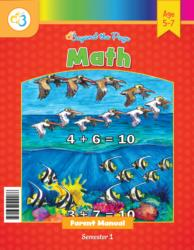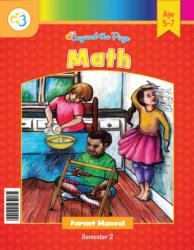# Common Core Alignment

CCSS.Math.Content.1.OA.8 - Determine the unknown whole number in an addition or subtraction equation relating three whole numbers. For example, determine the unknown number that makes the equation true in each of the equations 8 + ? = 11, 5 = � – 3, 6 + 6 = �.

## 5: MathUnit 3: Addition and Subtraction to 10
Lesson 3: Getting on Board with AdditionUnit 5: Addition and Subtraction to 20
Lesson 1: Addition and Subtraction Review
Lesson 6: Subtracting Within 20
Lesson 7: Problem Solving
Final Project: Numbers Are Missing!